## Calculating Amount of Days from First Entry

by Nicholas Bowskill

In this blog we will use an LOD to calculate the first entry date of purchase of products in the Superstore data set and then use this LOD to calculate the running sum of sales over amount of days after the first sale. This normalises the start of selling for products, so rather than starting their sum of sales from a certain date they start their sales from day 0, which make it much easier to compare how they are selling.

To start with we will create a Calculated Field to find the first order date of each product. We will want to use a FIXED LOD calculation, we fix the calculation on Product ID so that the Calculated Field will do the calculation for each product id and the calculation will be MIN([Order Date]). This LOD will then search the records in the data set for each product id and find the minimum date, or first date, that the product was ordered. In Tableau the calculation should look like: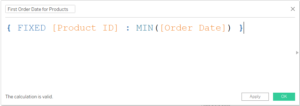We can now use this Calculated Field as a base in DATEDIFF calculations. To create the Calculated Field that will give us the amount of days each order is from the first order of that product we will find the difference in days between Order Date and our LOD, in this case First Order Date for Products. So the Calculated Field will be DATEDIFF( ‘day’ , [First Order Date for Products] , [Order Date] ), or in Tableau is: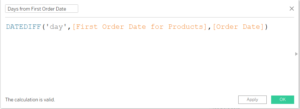As we don’t want to be aggregating the days from first order date, we need to convert the new Calculated Field, here called Days from First Order Date, into a continuous dimension. This can be done by dragging the Calculated Field from Measures into Dimensions in the tab on the left hand side of the screen and then right clicking on the pill and clicking Convert to Continuous.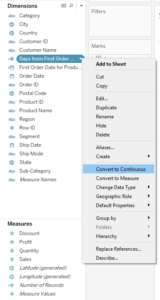Now the Days from First Order Date pill can be dragged onto the columns shelf and the measure that is wanted to be shown, here Sales, dragged onto the rows shelf, then add Product ID to the details card. The resulting graph that Tableau produces should like like this: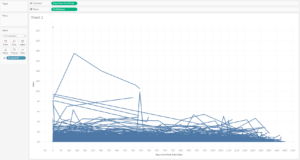This is now showing the sales for each product on each day that it has sold after it was first sold. If day of the Order Date was put on the column shelf instead the same lines would be shown for each product but they would all start at different points, whichever date they were first sold on.

It was said in the intro to this blog that we were wanting to see the running sum of sales for the products, as this is a useful comparison in seeing what sells well, so we shall add a table calculation to the SUM([Sales]). Running Total is a Quick Table Calculation option so we can use this; right click on the SUM([Sales]) pill in the rows shelf, go down to Quick Table Calculations and the select ‘Running Total‘: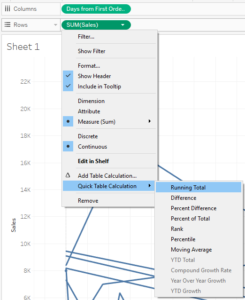We now get a view like: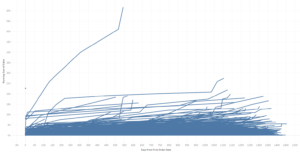And we can see that there is one product that is making us a lot of sales, but either has not been on sale for as like as the others (only 543 days) or has stopped being sold recently. As we probably don’t know the Product Id’s off the top of our head, we can drag the Product Name dimension onto the Tooltip marks card and then see that it is a Canon Advanced Copier that is selling so much.

From this point on many things can be done with this view; the lines could be made really thin to make it a little less messy, the highlight function can highlight certain products, the amount of days axis shortened to a certain amount (to see how many sold in the first 100 days perhaps) and other formatting. Or a different view could be made such as percent difference from start or amount of profit from start shown, with the initial LOD for finding the first order date the ‘days from first order’ world is now your oyster!Nicholas Bowskill

Fri 01 Feb 2019

Sun 20 Jan 2019

Sat 19 Jan 2019

Tue 08 Jan 2019